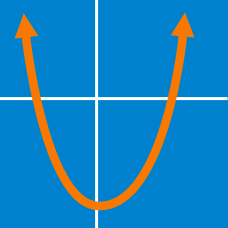Algebra

# Multiplying Polynomials - Quadratics

Which of the following is the expansion of

$x(x+4)?$

Which of the following is the expansion of

$(x+3)(x+4) ?$

It is given that

$( 3x+9)(6x+8) = Ax^2 + Bx + C$

is an algebraic identity. What is the value of $B$?

Simplify

$(3 x^2 + x - 4)+(6 x^2 - 9x + 2).$

What is the product of all the coefficients in the expansion of the expression $\left(\frac{1}{5}x-9\right)\left(\frac{1}{3}x-5\right) ?$

×